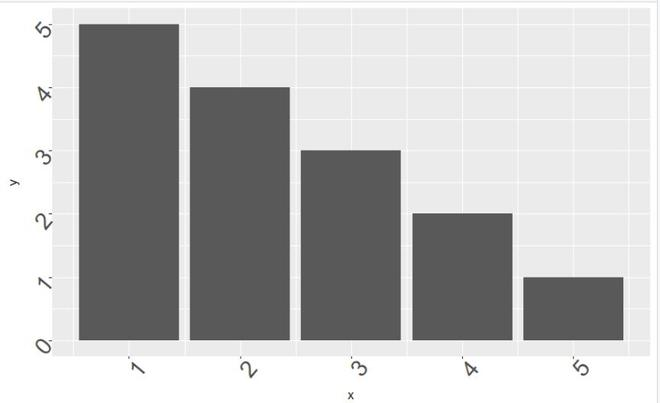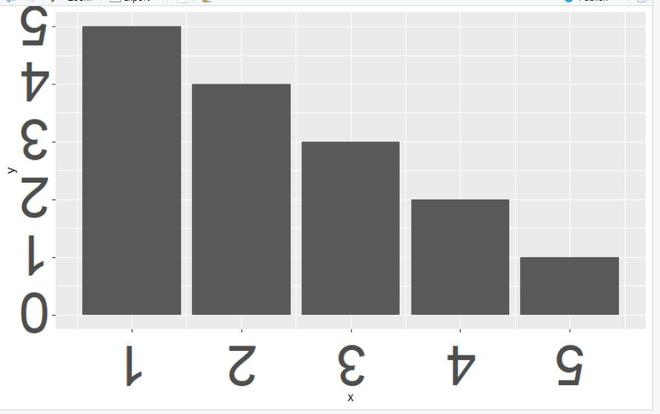Related Articles

# Changing Font Size and Direction of Axes Text in ggplot2 in R

• Last Updated : 06 Jun, 2021

In this article, we will discuss how to change the font size and the direction of the axis text using the ggplot2 plot in R Programming language.

For both of the requirement theme() function is employed. After plotting a regular graph, simply adding theme() with appropriate values will get the job done.

theme() function:

Use of this function is a powerful way to customize the non-data components of your plots: i.e. titles, labels, fonts, background, gridlines, and legends. This function can also be used to give plots a consistent customized look.

Syntax:

theme (line, text, axis.title,axis.text)

• Parameter:
• line: all line elements (element_line())
• text: all text elements (element_text())
• axis.title: labels of axes (element_text()). Specify all axes’ labels (axis.title)
• axis.text: tick labels along axes (element_text()). Specify all axis tick labels (axis.text)

To change text size and angle, theme() is called with axis.text set to appropriate values, this attribute as the name suggests acts upon axis text. To this element_text() function is called with its attribute- size and angle, set to a required value.

Syntax:

theme(axis.text = element_text(size, angle) )

Example:

## R

 `library``(``"ggplot2"``)   `` ` `gfg_data<-``data.frame``(x=``c``(1,2,3,4,5),y=``c``(5,4,3,2,1))`` ` `gfg_plot<-``ggplot``(data=gfg_data, ``aes``(x, y)) +``  ``geom_bar``(stat=``"identity"``)`` ` `gfg_plot + ``theme``(axis.text = ``element_text``(size = 20, angle=50)) `

Output:Example:

## R

 `library``(``"ggplot2"``)   `` ` `gfg_data<-``data.frame``(x=``c``(1,2,3,4,5),y=``c``(5,4,3,2,1))`` ` `gfg_plot<-``ggplot``(data=gfg_data, ``aes``(x, y)) +``  ``geom_bar``(stat=``"identity"``)`` ` `gfg_plot + ``theme``(axis.text = ``element_text``(size = 50, angle=180)) `

Output:My Personal Notes arrow_drop_up